MAT331 homework problems
20.
(expires 8 May)     Write a procedure in Maple that counts the frequency of letters in a string of text. For example, here is what it looks like when I use mine:

freqs("time flies like an arrow, fruit flies like a bananna.");

[[" ",9], ["i",6], ["a",6], ["e",5], ["n",4], ["l",4], ["r",3], ["f",3], ["t",2], ["s",2], ["k",2], ["w",1], ["u",1], ["o",1], ["m",1], ["b",1], [",",1], [".",1]]

In the above phrase, there are 9 spaces, 6 each of the letters i'' and a'', e'' appears 5 times, and so on. [Hint: I found it useful to group identical letters in the text using Implode(sort(Explode(text)) , but you might not.]

21.
(expires 8 May)     The string below was encrypted using an affine cipher on the 27 letter alphabet  abcdefghijklmnopqrstuvwxyz'' (there is a space in the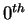position.) Decrypt it.

fmw segjaweoouanerj a ceyqrype aswaheoaqbrqabeafrua eeaojerf afmjeayperjpu

Hint: this phrase follows the the typical pattern in English where there are as many spaces as words (so spaces are very common), and the letter e'' is also very common. You can use the technique described in chapter 4 of the notes, section 7.3.

22.
(expires 8 May)     Recall that a Vignère cipher can be interpreted as a Caesar-like cipher on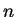-vectors, whereis the length of the key phrase. Can every affine encipherment on digraphs (two-character codes) be interpreted as an affine matrix encipherment on 2-vectors? That is, suppose I encode a message by affine enciphering on digraphs. Can I always get the same crypttext from the same plaintext using an affine matrix enciphering (using a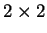matrix) on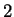-vectors? If your answer is yes, prove it. If no, give a counter-example that cannot be so interpreted.

EqOG3u0qaJTX.ccwlqa9UhjkFKRT8a)CWn1rs3u(PsfK8OPsNde2 9OW8OtURd(qdUx!nDo4R2

Hint: the above used an affine matrix cipher on 2-vectors, with the 67-letter alphabet

1234567890.! ()ABCDEFGHIJKLMNOPQRSTUVWXYZabcdefghijklmnopqrstuvwxyz

You should be able to guess what the first 20 letters of the message are.

24.
(expires 8 May)     Modify the AffineMatEncode routine we wrote in class so that you can use a text string as a key instead of a matrix and a vector. For example, if the phrase is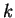characters long, the key should be an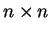matrix and an-vector, where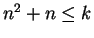. The elements of the key matrix and vector should be the numerical equivalents of the characters in the key phrase. Do something sensible with any extra letters (that is, if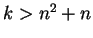). Be sure to check that the resulting matrix is nonsingular.

MAT 331 2002-04-27